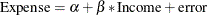# Introduction to Survey Sampling and Analysis Procedures

### Survey Data Analysis

You can use the SURVEYMEANS and SURVEYREG procedures to estimate population values and perform regression analyses for survey data. The following example briefly shows the capabilities of these procedures. For more information, see Chapter 99: The SURVEYMEANS Procedure, and Chapter 101: The SURVEYREG Procedure.

The following PROC SURVEYMEANS statements estimate the total income and living expenses for the survey population based on the data from the stratified sample design:

   proc surveymeans data=HHSample sum median;
var Income Expense;
strata State Region;
weight Weight;
run;


The PROC SURVEYMEANS statement invokes the procedure, and the DATA= option names the SAS data set HHSample as the input data set to be analyzed. The keywords SUM and MEDIAN request estimates of population totals and medians.

The VAR statement specifies the two analysis variables Income and Expense. The STRATA statement names the stratification variables State and Region. The WEIGHT statement specifies the sampling weight variable Weight.

You can use PROC SURVEYREG to perform regression analysis for survey data. Suppose that, in order to explore the relationship between household income and living expenses in the survey population, you choose the following linear model:The following PROC SURVEYREG statements fit this linear model for the survey population based on the data from the stratified sample design:

   proc surveyreg data=HHSample;
strata State Region ;
model  Expense = Income;
weight Weight;
run;


The STRATA statement names the stratification variables State and Region. The MODEL statement specifies the model, with Expense as the dependent variable and Income as the independent variable. The WEIGHT statement specifies the sampling weight variable Weight.